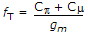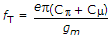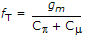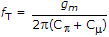# Electronics and Communication Engineering - Electronic Devices and Circuits

1.

Assertion (A): Hall effect is used to find the type of semiconductor.

Reason (R): When a specimen of semiconductor carrying current I lies in a magnetic field the force on electrons and holes is in opposite directions.

Both A and R are true and R is correct explanation of A
Both A and R are true but R is not a correct explanation of A
A is true but R is false
A is false but R is true
Explanation:
No answer description is available. Let's discuss.

2.
The fT of a BJT is related to its gm, Cp and Cμ as follows.Explanation:
No answer description is available. Let's discuss.

3.
When a p-n junction is forward biased. The width of depletion layer decreases.
True
False
Explanation:
No answer description is available. Let's discuss.

4.
In which of the following case the rating of the transformer to deliver 100 watts of d.c. power to a load, will be least?
Half wave rectifier
Full wave rectifier
Bridge type full wave rectifier
Three phase full wave rectifier